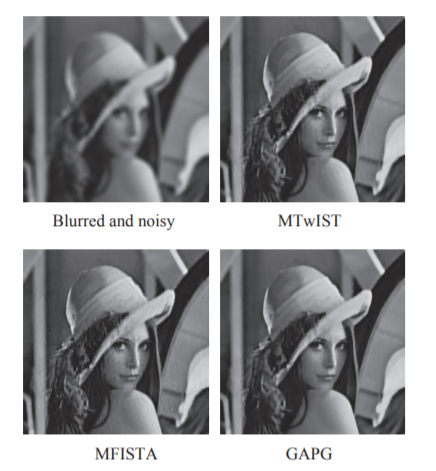# A Generalized Accelerated Proximal Gradient Approach for Total Variation-Based Image Restoration### Abstract

This paper proposes a generalized accelerated prox- imal gradient (GAPG) approach for solving total variation (TV) based image restoration problems. The GAPG algorithm generalizes the original APG algorithm by replacing the Lipschitz constant with an appropriate positive definite matrix, resulting in faster convergence. For TV-based image restoration problems, we further introduce two auxiliary variables that approximate the partial derivatives. Constraints on the variables can be easily imposed without modifying the algorithm much, and the TV regularization can be either isotropic or anisotropic. Compared with the recently developed APG-based methods for TV-based image restoration, i.e., monotone version of the two- step iterative shrinkage/thresholding algorithm (MTwIST) and monotone version of the fast iterative shrinkage/thresholding algorithm (MFISTA), our GAPG is much simpler as it does not require to solve an image denoising subproblem. Moreover, the convergence rate of O(k^{-2}) is maintained by our GAPG, where k is the number of iterations, the cost of each iteration in GAPG is also lower. As a result, in our experiments our GAPG approach can be much faster than MTwIST and MFISTA. The experiments also verify that our GAPG converges faster than the original APG and MTwIST when they solve identical problems.

Type
Publication
Transactions on Image Processing